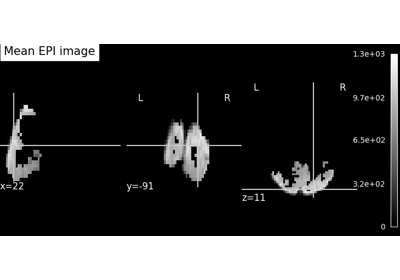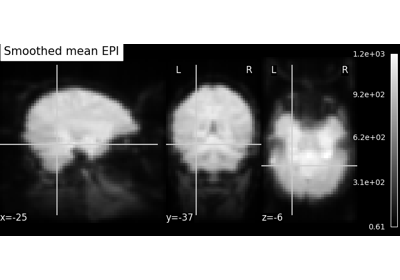Note

This page is a reference documentation. It only explains the function signature, and not how to use it. Please refer to the user guide for the big picture.

# nilearn.plotting.plot_epi#

nilearn.plotting.plot_epi(epi_img=None, cut_coords=None, output_file=None, display_mode='ortho', figure=None, axes=None, title=None, annotate=True, draw_cross=True, black_bg=True, colorbar=False, cbar_tick_format='%.2g', cmap=<matplotlib.colors.LinearSegmentedColormap object>, vmin=None, vmax=None, **kwargs)[source]#

Plot cuts of an EPI image (by default 3 cuts: Frontal, Axial, and Lateral)

Parameters:
epi_imga nifti-image like object or a filename, optional

The EPI (T2*) image.

cut_coordsNone, a `tuple` of `float`, or `int`, optional

The MNI coordinates of the point where the cut is performed.

• If `display_mode` is ‘ortho’ or ‘tiled’, this should be a 3-tuple: `(x, y, z)`

• For `display_mode == 'x'`, ‘y’, or ‘z’, then these are the coordinates of each cut in the corresponding direction.

• If `None` is given, the cuts are calculated automatically.

• If `display_mode` is ‘mosaic’, and the number of cuts is the same for all directions, `cut_coords` can be specified as an integer. It can also be a length 3 tuple specifying the number of cuts for every direction if these are different.

Note

If `display_mode` is ‘x’, ‘y’ or ‘z’, `cut_coords` can be an integer, in which case it specifies the number of cuts to perform.

output_file`str`, or None, optional

The name of an image file to export the plot to. Valid extensions are .png, .pdf, .svg. If `output_file` is not None, the plot is saved to a file, and the display is closed.

display_mode{‘ortho’, ‘tiled’, ‘mosaic’,’x’,’y’, ‘z’, ‘yx’, ‘xz’, ‘yz’}, optional

Choose the direction of the cuts:

• ‘x’: sagittal

• ‘y’: coronal

• ‘z’: axial

• ‘ortho’: three cuts are performed in orthogonal directions

• ‘tiled’: three cuts are performed and arranged in a 2x2 grid

• ‘mosaic’: three cuts are performed along multiple rows and columns

Default=’ortho’.

figure`int`, or `matplotlib.figure.Figure`, or None, optional

Matplotlib figure used or its number. If `None` is given, a new figure is created.

axes`matplotlib.axes.Axes`, or 4 tupleof `float`: (xmin, ymin, width, height), optional

The axes, or the coordinates, in matplotlib figure space, of the axes used to display the plot. If `None`, the complete figure is used.

title`str`, or None, optional

The title displayed on the figure. Default=None.

annotate`bool`, optional

If `annotate` is `True`, positions and left/right annotation are added to the plot. Default=True.

draw_cross`bool`, optional

If `draw_cross` is `True`, a cross is drawn on the plot to indicate the cut position. Default=True.

black_bg`bool`, or ‘auto’, optional

If `True`, the background of the image is set to be black. If you wish to save figures with a black background, you will need to pass facecolor=’k’, edgecolor=’k’ to `matplotlib.pyplot.savefig`. Default=True.

colorbarboolean, optional

If True, display a colorbar on the right of the plots. Default=False.

cbar_tick_format: str, optional

Controls how to format the tick labels of the colorbar. Ex: use “%i” to display as integers. Default is ‘%.2g’ for scientific notation.

cmap`matplotlib.colors.Colormap`, or `str`, optional

The colormap to use. Either a string which is a name of a matplotlib colormap, or a matplotlib colormap object. Default=`plt.cm.nipy_spectral`.

vmin`float`, optional

Lower bound of the colormap. If `None`, the min of the image is used. Passed to `matplotlib.pyplot.imshow`.

vmax`float`, optional

Upper bound of the colormap. If `None`, the max of the image is used. Passed to `matplotlib.pyplot.imshow`.

Notes

Arrays should be passed in numpy convention: (x, y, z) ordered.

## Examples using `nilearn.plotting.plot_epi`#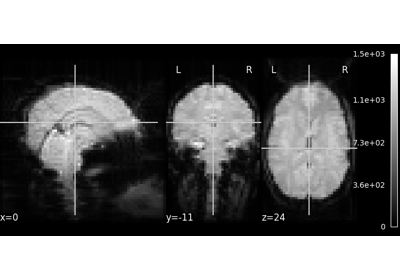A introduction tutorial to fMRI decoding

A introduction tutorial to fMRI decoding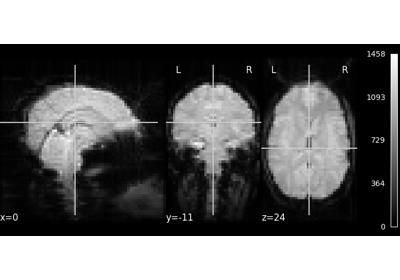NeuroImaging volumes visualization

NeuroImaging volumes visualization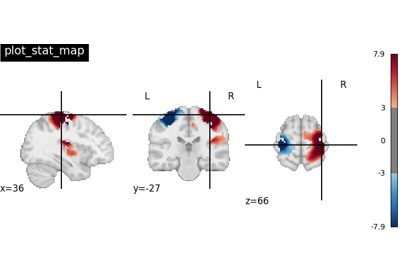Plotting tools in nilearn

Plotting tools in nilearn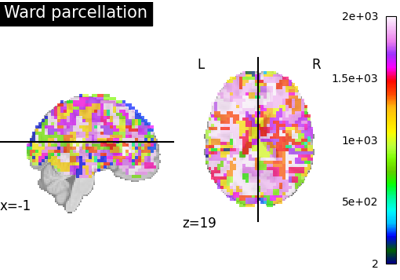Clustering methods to learn a brain parcellation from fMRI

Clustering methods to learn a brain parcellation from fMRI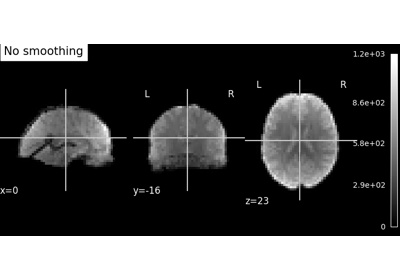Smoothing an image

Smoothing an image